Journal of Integer Sequences, Vol. 16 (2013), Article 13.6.4

## On the Representation of the Natural Numbers as the Sum of Three Terms of the Sequence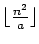### Bakir Farhi Department of Mathematics University of Béjaia Béjaia Algeria

Abstract:

In this note, we study the representation of a natural number as the sum of three natural numbers having the form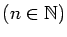, where a is a fixed positive integer and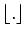denotes the integer-part function. By applying Gauss's triangular number theorem, we show that every natural number is the sum of three numbers of the form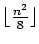. And by applying Legendre's theorem, we show that every natural number is the sum of three numbers of the form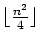and that every natural number N ≢ 2 (mod 24) is the sum of three numbers of the form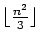. On the other hand, we show that every even natural number is the sum of three numbers of the form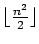. We also propose two conjectures on the subject.

Full version:  pdf,    dvi,    ps,    latex

Received January 21 2013; revised versions received January 31 2013; May 25 2013; July 3 2013. Published in Journal of Integer Sequences, July 4 2013.

Return to Journal of Integer Sequences home page